# Ratio of volumes

If the heights of two cylindrical drums are in the ratio 7:8 and their base radii are in the ratio 4:3. What is the ratio of their volumes?

Correct result:

p =  14:9

#### Solution: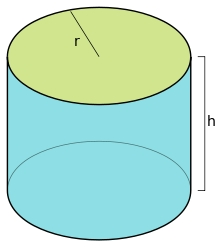Our examples were largely sent or created by pupils and students themselves. Therefore, we would be pleased if you could send us any errors you found, spelling mistakes, or rephasing the example. Thank you!

Please write to us with your comment on the math problem or ask something. Thank you for helping each other - students, teachers, parents, and problem authors.Tips to related online calculators
Need help calculate sum, simplify or multiply fractions? Try our fraction calculator.
Check out our ratio calculator.
Tip: Our volume units converter will help you with the conversion of volume units.

#### You need to know the following knowledge to solve this word math problem:

We encourage you to watch this tutorial video on this math problem:

## Next similar math problems:

• Equilateral coneWe pour so much water into a container that has the shape of an equilateral cone, the base of which has a radius r = 6 cm, that one-third of the volume of the cone is filled. How high will the water reach if we turn the cone upside down?
• Mixing 5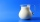Carlos mixed 4/15 of chocolate syrup with 1/2 of milk. Determine the reasonable estimate of the total amount of liquid
• Final exam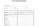At the final exam, the student answers from three areas, which are evaluated in a ratio of 1: 2: 2. What grade will John receive if he answered as follows: 3,1,2.
• The plan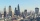The plan of the housing estate is in three scales 1: 5000,1: 10000,1: 15000. The distance between two points on a plan with a scale of 1: 10000 is 12 cm. What is this distance on the other two plans? What is this distance?
• CoinsThe money - coins are minted from the hardest bronze, which contains copper and tin in a ratio of 41: 9. How much copper and tin are in 2kg of bronze money?How many times does the surface of a sphere decrease if we reduce its radius twice?
• Divide in ratioLine segment AB 12 cm long divide in a ratio of 5: 3. How long are the individual parts?
• Ratio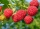Alena collected 7.8 kg of blueberries, 2.6 kg of blackberries, and 3.9 kg of cranberries. Express the ratio in the smallest natural numbers in this order.
• SummandsWe want to split the number 110 into three summands so that the first and the second summand are in the ratio 4: 5, and the third with the first are in ratio 7: 3. Calculate the smallest of the summands.
• Two ribbonsThe total length of two ribbons is 13 meters. If one ribbon is 7 and 5/8 meters long, what is the length of the other ribbon.
• Can of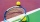Can of tennis balls contains 3 balls per can and cost \$7 how much will it cost for 36 tennis balls?2 and 1 8th plus 1 and 1 3rd =In the triangle DEF is DE = 21cm, EF = 14.7cm, DF = 28cm. The triangle D´E´F´ is similar to the triangle DEF. Calculate the lengths of the sides of the triangle D´E´F´ if the similarity coefficient is one-seventh.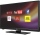How many hours the Andersons watched TV in all Wednesday 3/1 hr Thursdays 2/3 hr Friday 4/5 hr Saturday 3/4 hr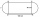Calculate the length of a semicircle with a radius of 6cm.Josh is at Enzo's pizza palace. He can sit at a table with 5 of his friends or at a different table with seven of his friends. The same size pizza is shared equally among the people at each table. At which table should Josh sit to get more pizza? (write a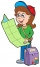The tourist walked a quarter of the way on the first day, a third of the rest on the second day, and 20 km on the last day. How many km did he walk in three days?